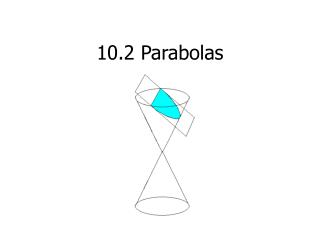DownloadDownload Presentation10.2 Parabolas

# 10.2 Parabolas

Download Presentation## 10.2 Parabolas

- - - - - - - - - - - - - - - - - - - - - - - - - - - E N D - - - - - - - - - - - - - - - - - - - - - - - - - - -
##### Presentation Transcript

1. 10.2 Parabolas

2. Objective • To determine the relationship between the equation of a parabola and its focus, directrix, vertex, and axis of symmetry. • To graph a parabola

3. Definition • A set of points equidistant from a fixed point (focus) and a fixed line (directrix).

4. The midpoint between the focus and the directrix is called the vertex. • The line passing through the focus and the vertex is called the axis of the parabola. • A parabola is symmetric with respect to its axis.

5. p is the distance from the vertex to the focus and from the vertex to the directrix.

6. Vertical

7. General Form If p > 0 opens up, if p < 0 opens down

8. Vertex: (h, k) • Focus: (h, k + p) • Directrix: y = k – p • Axis of symmetry: x = h • If the vertex is at the origin (0, 0), the equation is:

9. Horizontal parabola

10. General Form If p > 0 opens right, if p < 0 opens left

11. Vertex: (h, k) • Focus: (h + p, k) • Directrix: x = h-p • Axis of symmetry: y = k

12. Example 1 • Find the standard equation of the parabola with vertex (3, 2) and focus (1, 2)

13. Example2Finding the Focus of a Parabola • Find the focus of the parabola given by

14. Example 3Finding the Standard Equation of a Parabola • Find the standard form of the equation of the parabola with vertex (1, 3) and focus (1, 5)

15. Example 4 • opens: p = • vertex focus • directrix axis of symmetry

16. Application • A line segment that passes through the focus of a parabola and has endpoints on the parabola is called a focal chord. The focal chord perpendicular to the axis of the parabola is called the latus retum.

17. A line is tangent to a parabola at a point on the parabola if the line intersects, but does not cross, the parabola at the point. • Tangent lines to parabolas have special properties related to the use of parabolas in constructing reflective surfaces.

18. Reflective Property of a Parabola • The Tangent line to a parabola at a point P makes equal angles with the following two line: • The line passing through P and the focus • The axis of the parabola.

19. Example 5Finding the Tangent Line at a point on a Parabola • Find the equation of the tangent line to the parabola given by • At the point (1, 1)